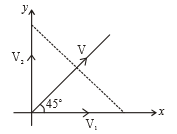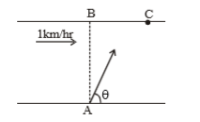Three particles moving with constant velocities  and V respectively as given in the figure. After some time all three particles are in the same line, then relation among  and V is1.

2.

3.

4.

Concept Questions :-

Speed and velocity
High Yielding Test Series + Question Bank - NEET 2020

Difficulty Level:

A river is flowing with a speed of 1 km/hr. A swimmer wants to go to point 'C' starting from 'A'. He swims with a speed of 5 km/hr, at an angle $\theta$ w.r.t. the river. If AB=BC=400m. Then-(1) time taken by the man is 12 min

(2) time taken by the man is 8 min

(3) the value of $\theta$ is 45$°$

(4) the value of $\theta$ is 53$°$

Concept Questions :-

Relative motion
High Yielding Test Series + Question Bank - NEET 2020

Difficulty Level:

A body is thrown horizontally with a velocity $\sqrt{2gh}$ from the top of a tower of height h. It strikes the level ground through the foot of the tower at a distance x from the tower. The value of x is:

(1) h

(2) $\frac{h}{2}$

(3) 2h

(4) $\frac{2h}{3}$

Concept Questions :-

Projectile motion
High Yielding Test Series + Question Bank - NEET 2020

Difficulty Level:

A particle starts from the origin at t=0 and moves in the x-y plane with constant acceleration 'a' in the y direction. Its equation of motion is $y=b{x}^{2}$. The x component of its velocity (at t=0) is:

(1) variable

(2) $\sqrt{\frac{2a}{b}}$

(3) $\frac{a}{2b}$

(4) $\sqrt{\frac{a}{2b}}$

Concept Questions :-

Acceleration
High Yielding Test Series + Question Bank - NEET 2020

Difficulty Level:

A body projected with velocity u with an angle of projection $\theta$. Change in velocity after the time (t) from the projection is:

(1) gt

(2) $\frac{1}{2}g{t}^{2}$

(3) u sin$\theta$

(4) u cos$\theta$

Concept Questions :-

Projectile motion
High Yielding Test Series + Question Bank - NEET 2020

Difficulty Level:

A particle has initial velocity $\left(3\stackrel{^}{\mathrm{i}}+4\stackrel{^}{\mathrm{j}}\right)$ and has acceleration $\left(0.4\stackrel{^}{\mathrm{i}}+0.3\stackrel{^}{\mathrm{j}}\right)$. Its speed after 10 s

1. 7 units

2. $7\sqrt{2}$ units

3. 8.5 units

4. 10 units

Concept Questions :-

Uniformly accelerated motion
High Yielding Test Series + Question Bank - NEET 2020

Difficulty Level:

The gravity in space is given by . Two particles are simultaneously projected with velocity  and . Then, the ratio of their times of flight

1. 1:1

2. 1:2

3. 2:1

4. none

Concept Questions :-

Projectile motion
High Yielding Test Series + Question Bank - NEET 2020

Difficulty Level:

The x and y coordinates of a particle at any time t are given by $x=7t+4{t}^{2}$ and y = 5t, where x and y are in metre and t in seconds. The acceleration of particle at t = 5s is

(1) Zero

(2) 8 m/s2

(3) 20 m/s2

(4) 40 m/s2

Concept Questions :-

Acceleration
High Yielding Test Series + Question Bank - NEET 2020

Difficulty Level:

A boat is sent across a river in perpendicular direction with a velocity of 8 km/hr. If the resultant velocity of boat is 10 km/hr, then velocity of the river is :

(1) 10 km/hr

(2) 8 km/hr

(3) 6 km/hr

(4) 4 km/hr

Concept Questions :-

Relative motion
High Yielding Test Series + Question Bank - NEET 2020

Difficulty Level:

A boat moves with a speed of 5 km/h relative to water in a river flowing with a speed of 3 km/h and having a width of 1 km. The minimum time taken around a round trip is

(1) 5 min

(2) 60 min

(3) 20 min

(4) 30 min

Concept Questions :-

Relative motion